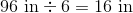# ISEE Middle Level Quantitative : How to find length of a line

## Example Questions

### Example Question #1 : How To Find Length Of A Line

Which is the greater quantity?

(a) 370 meters

(b) 3,700 centimeters

(a) and (b) are equal

(a) is the greater quantity

It is impossible to determine which is greater from the information given

(b) is the greater quantity

(a) is the greater quantity

Explanation:

One meter is equivalent to 100 centimeters, so 370 meters can be converted to centimeters by multiplying by 100: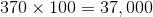.

370 meters are equal to 37.000 centimeters and is the greater quantity.

### Example Question #2 : How To Find Length Of A Line

Which is the greater quantity?

(a) 4.8 kilometers

(b) 4,800 meters

(b) is the greater quantity

It is impossible to determine which is greater from the information given

(a) is the greater quantity

(a) and (b) are equal

(a) and (b) are equal

Explanation:

One kilometer is equal to 1,000 meters, so convert 4.8 kilometers to meters by multiplying by 1,000: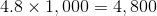meters

The two quantities are equal.

### Example Question #3 : How To Find Length Of A Line

A regular pentagon has perimeter 1 yard. Give the length of one side.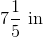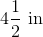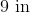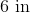Explanation:

A regular pentagon has five sides of equal length. The perimeter, which is the sum of the lengths of these sides, is one yard, which is equal to 36 inches. Therefore, the length of one side is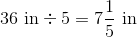.

### Example Question #4 : How To Find Length Of A Line

A regular hexagon has perimeter 8 feet. Give the length of one side.

12 inches

24 inches

18 inches

16 inches

16 inches

Explanation:

The perimeter can be converted from feet to inches by multiplying by conversion factor 12 {inches per foot):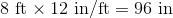A regular hexagon has six sides of equal length. Divide this perimeter by 6 to obtain the length of each side: